# sketch-a-day

#### coding a visual idea a day

Welcome! My name is Alexandre Villares and since January, 2018 I have been coding sketches everyday, publishing the source code in the same repository that stores this page, github.com/villares/sketch-a-day.

The results are mostly tentative, exploratory, and I don’t feel like they need to be relevant or meaningful on any particular day. The everyday practice leads to the emergence of ideas that I consider interesting, worthy of further exploration. Some of those have been added to selected work, this collection itself became valuable for me, and it is my pleasure to share it with anyone willing to explore coding as a creative and expressive medium.

Please do not hesitate to contact me regarding licenses to use my work, teaching opportunities, consulting or other projects. Moreover, I kindly invite you to subscribe to my newsletter, [sketch-mail]. If you appreciate what I have been doing, you may support my artistic work, research and open educational resources I publish on-line using gumroad.com/villares, PayPal or PIX at `46c37783-5edb-4f1c-b3a8-1309db11488c`.

Here are listed some of the tools I have been using:

• [py5] A new Processing Java + Python 3 amazing tool
• [pyp5js] initially a Python to p5js trancriptor, now a pyodide + p5js tool
• [pyscript] A new Python in the browser tool.
• [Py.Processing] Processing Python mode
• [Processing Java Processing Java or “standard” mode
• [p5.js] JavaScript library from the Processing Foundation
• [shoebot] Generate 2D vector graphics with Python
• [FreeCAD] A wonderful 3D modeling tool, CAD & more, Python infused.
• [p5py] A Python 3 implementation of Processing ideas (no Java needed)
• [flat] A generative infrastructure library for Python (via Allison Parrish’s Bezmerizing)
• [VPython] Python + glowscript gives you 3D on the browser
• [PySimpleGUI] Python GUIs for Humans

## 2023 | 2022 | 2021 | 2020 | 2019 | 2018

### sketch_2023_01_27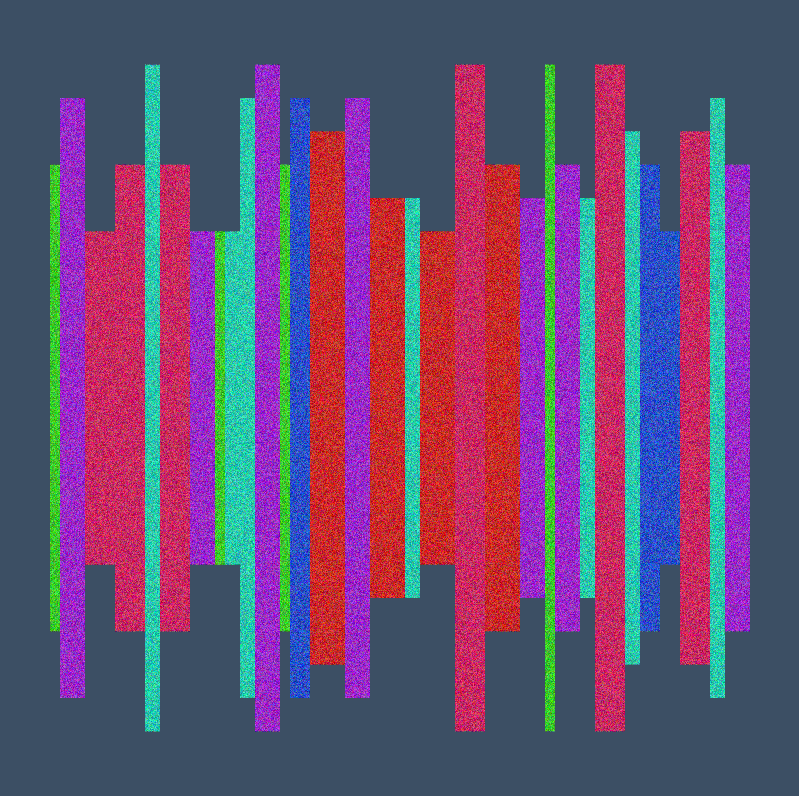Based on Tom Larrow’s experiment at: https://codeberg.org/TomLarrow/creative-coding-experiments/src/branch/main/x_0049/x_0049.py

### sketch_2023_01_26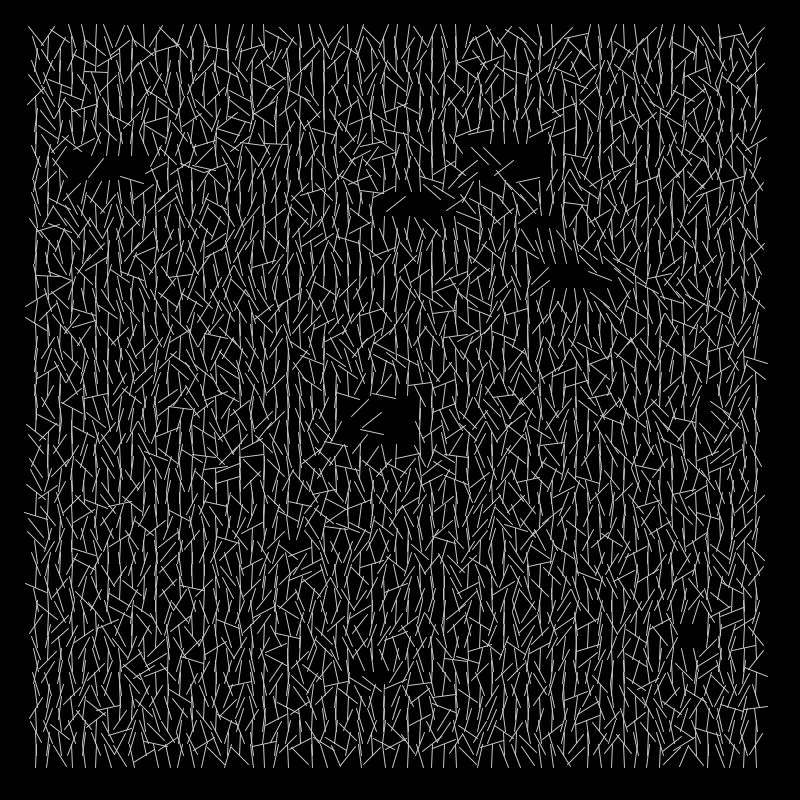d’aprés Vera Molnar “interruptions” (https://collections.vam.ac.uk/item/O1193775/interruptions-drawing-vera-molnar/)dapr

### sketch_2023_01_25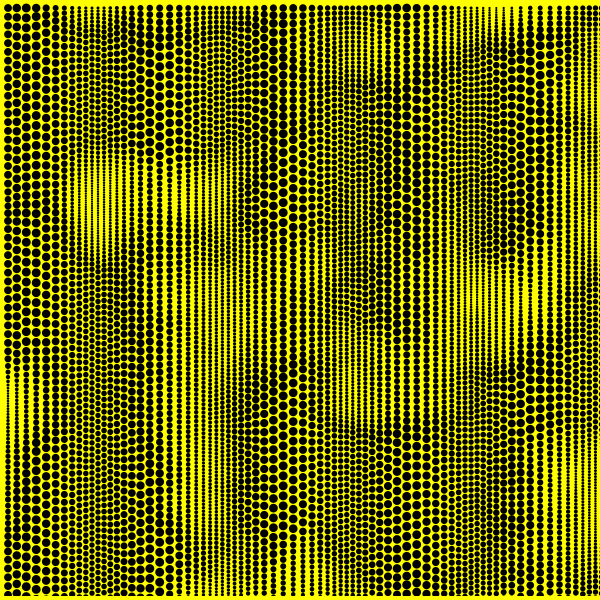### sketch_2023_01_24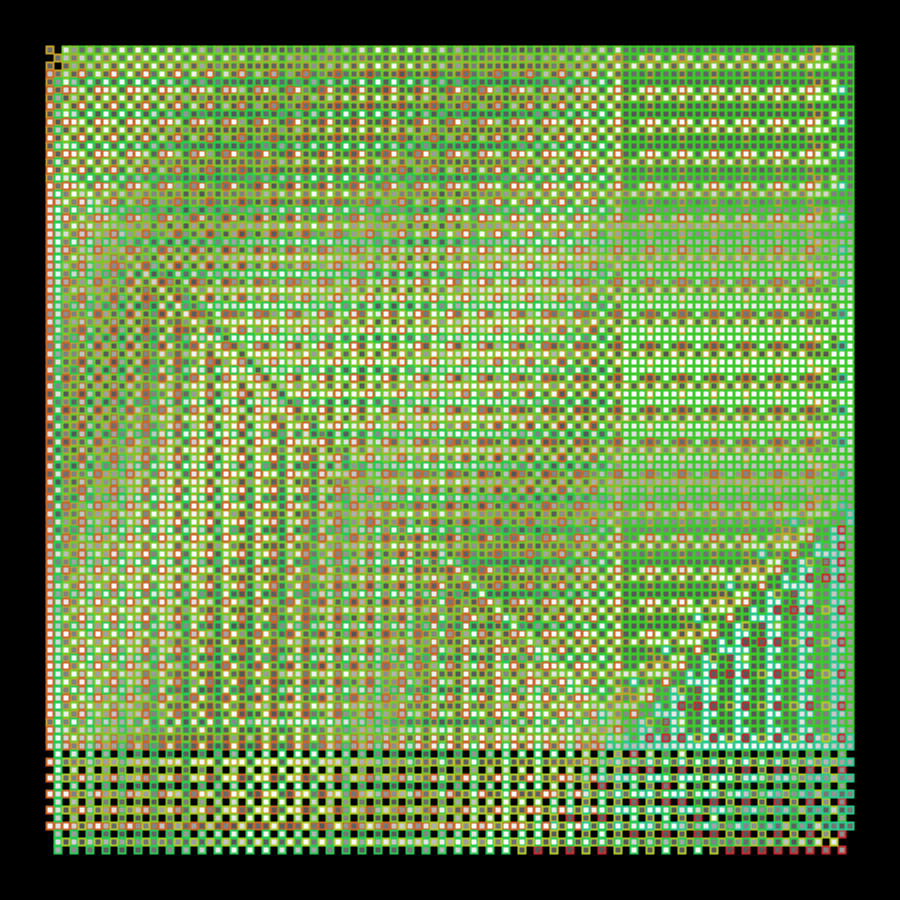### sketch_2023_01_23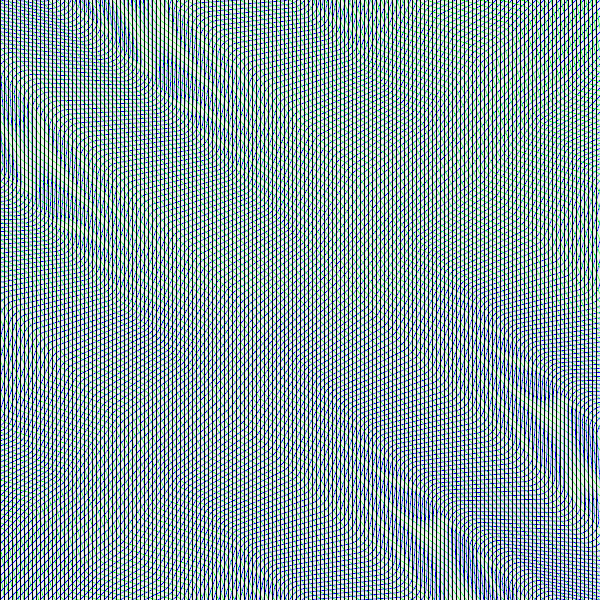### sketch_2023_01_22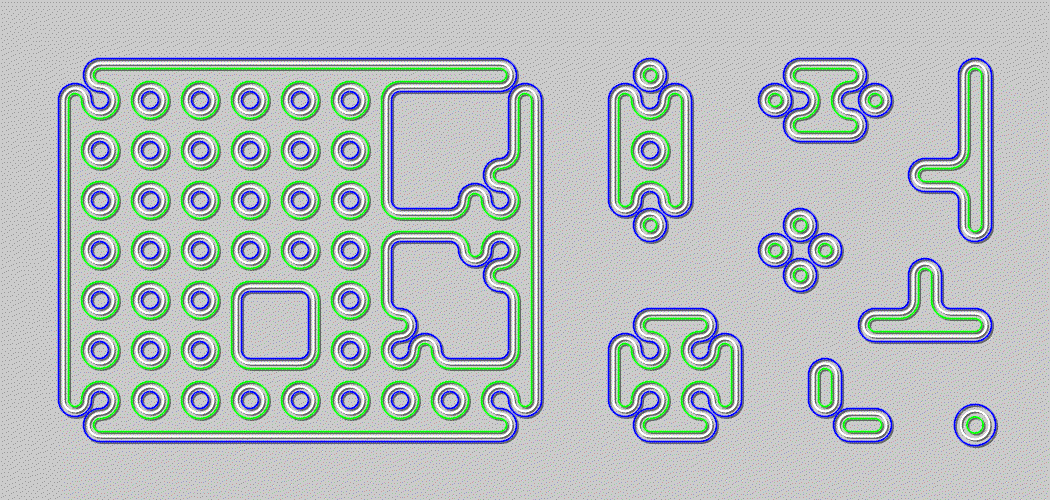### sketch_2023_01_21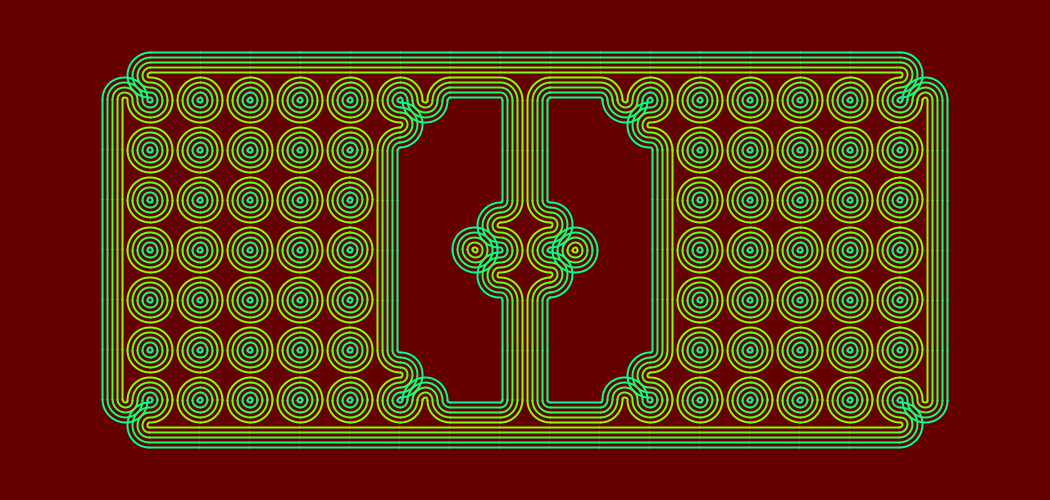### sketch_2023_01_20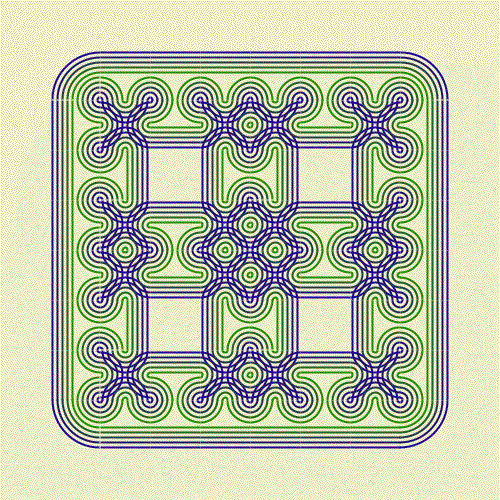### sketch_2023_01_19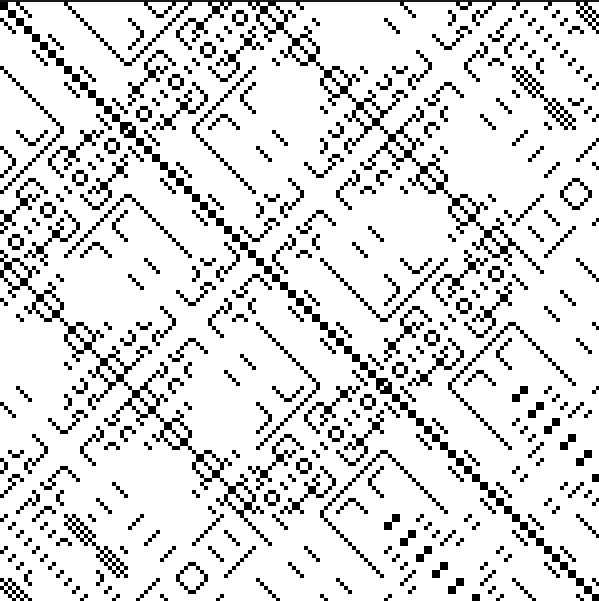### sketch_2023_01_18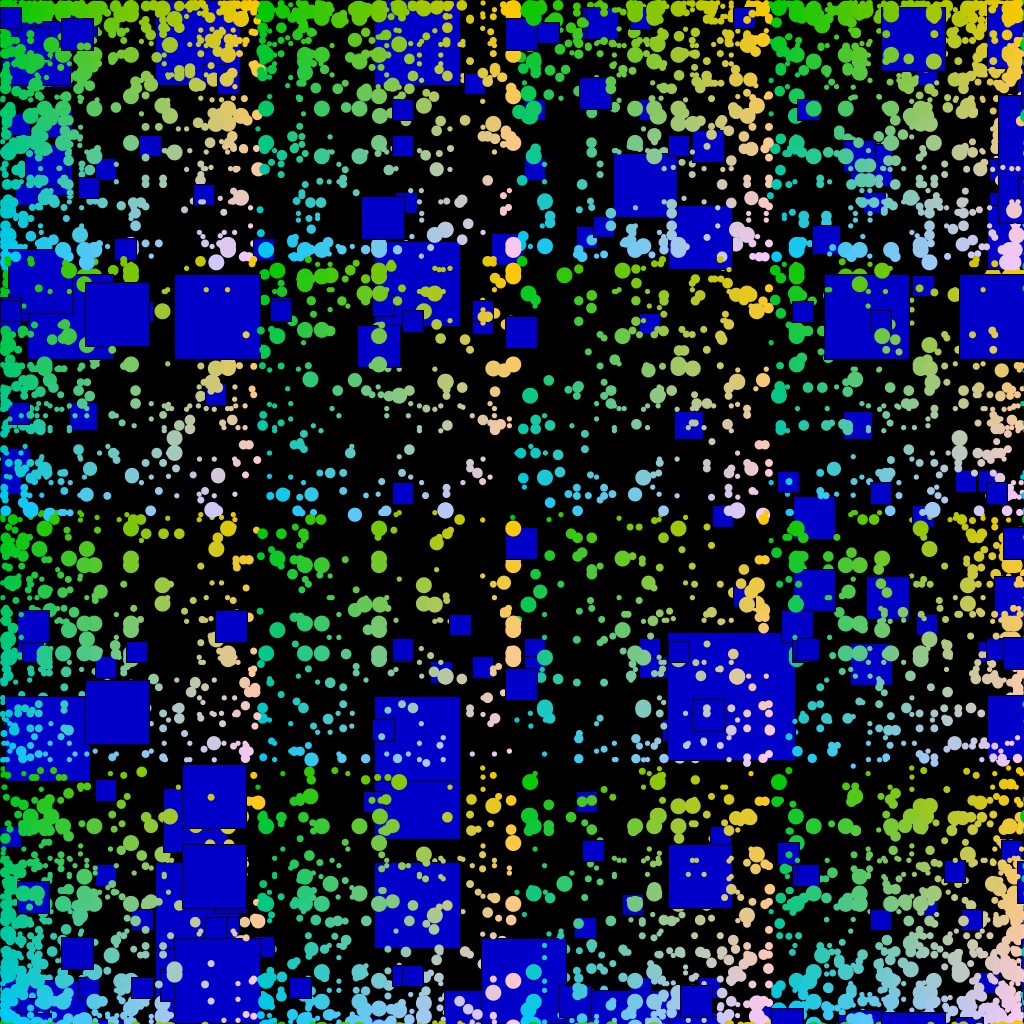### sketch_2023_01_17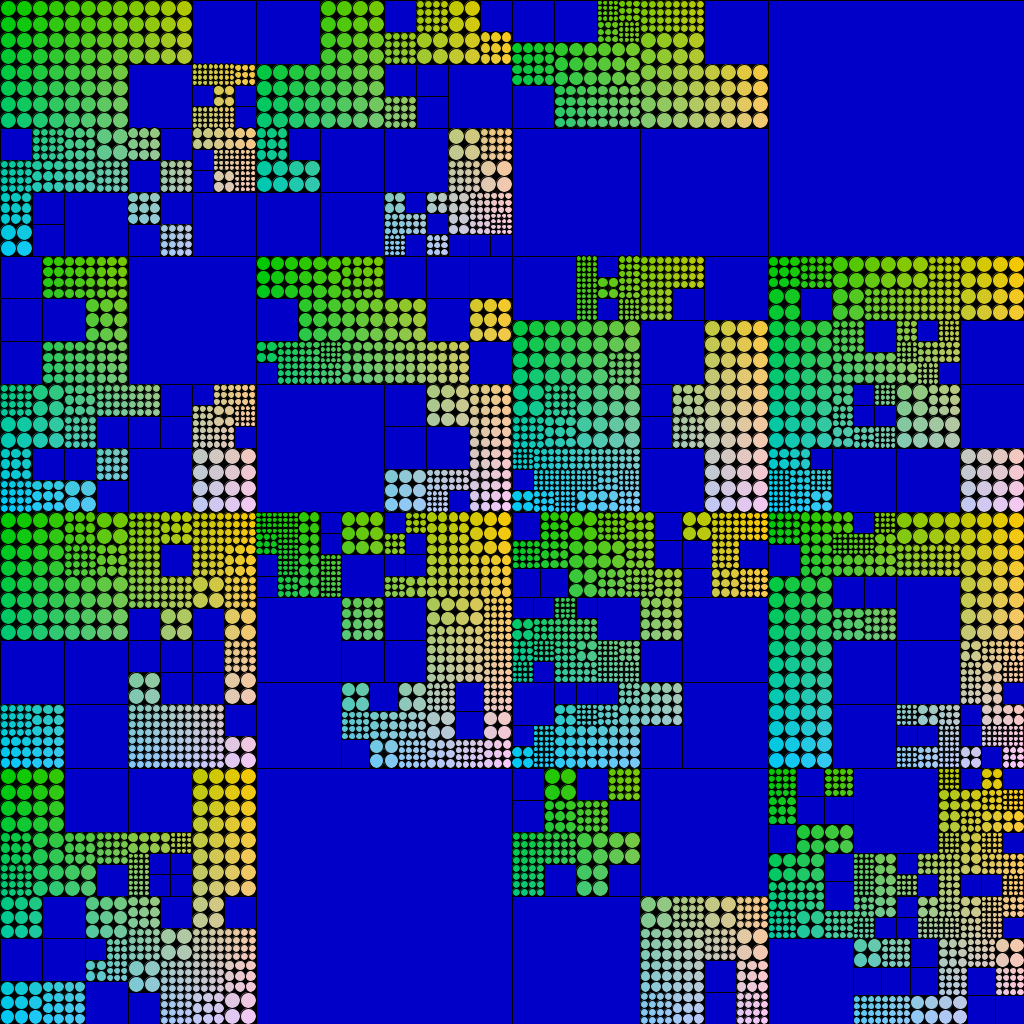### sketch_2023_01_16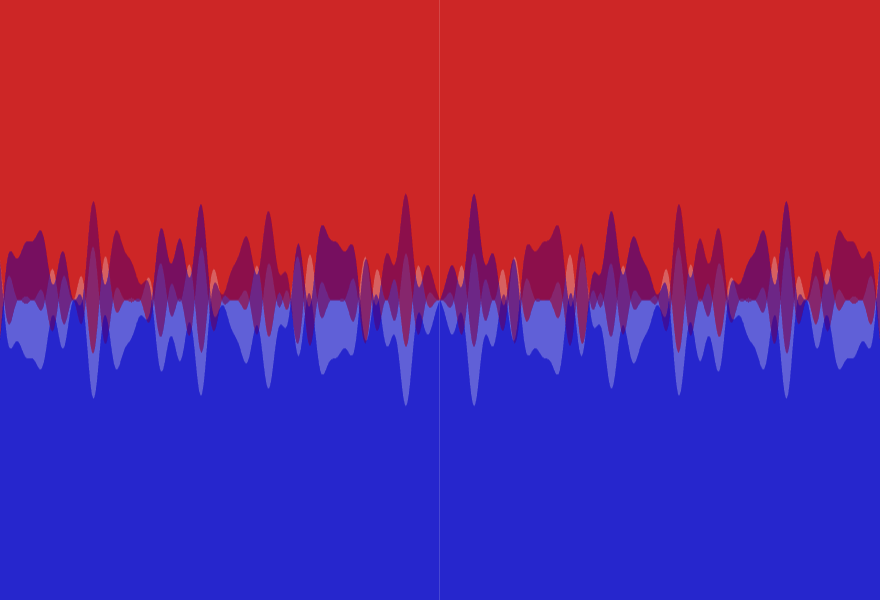### sketch_2023_01_15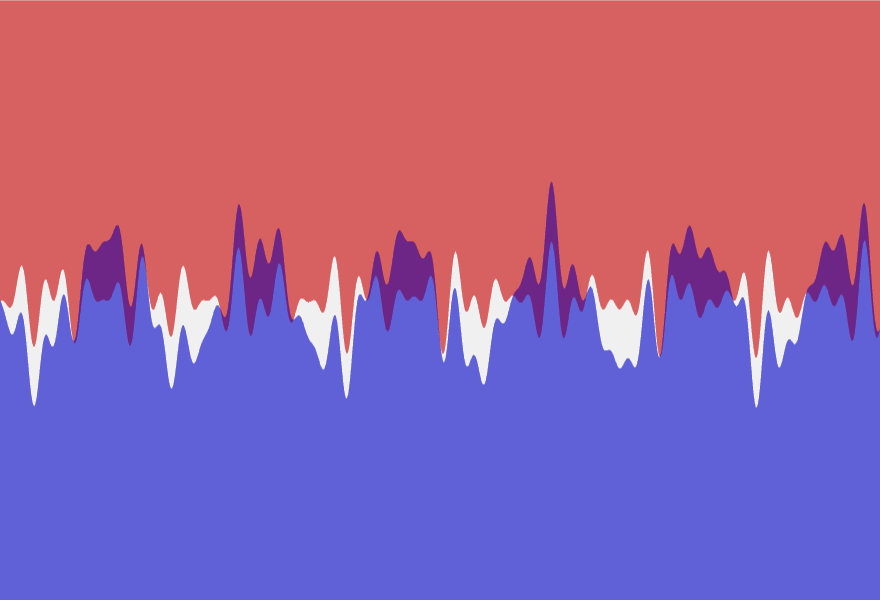### sketch_2023_01_14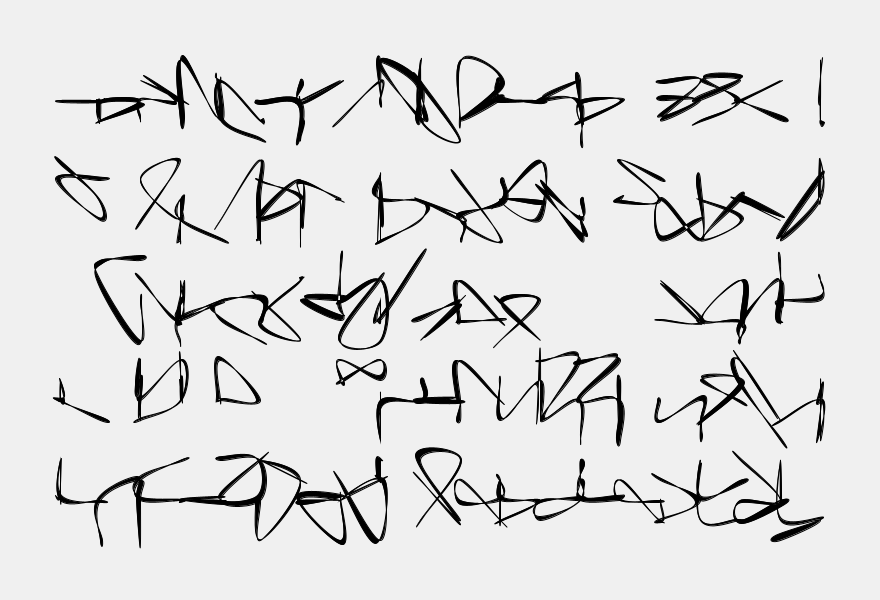### sketch_2023_01_13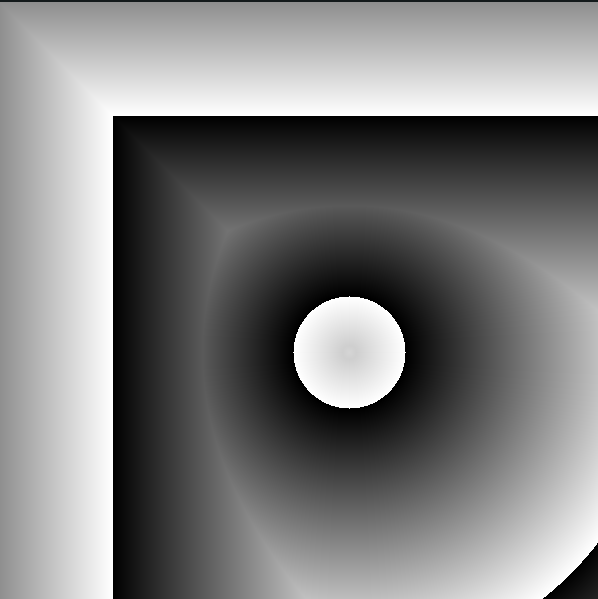### sketch_2023_01_12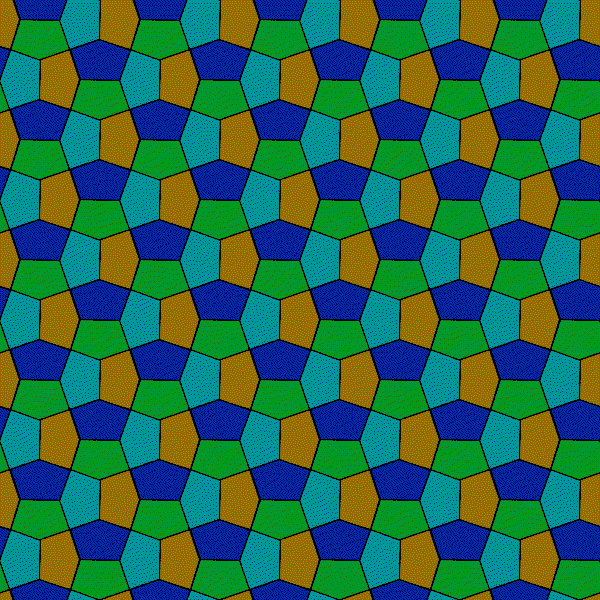### sketch_2023_01_11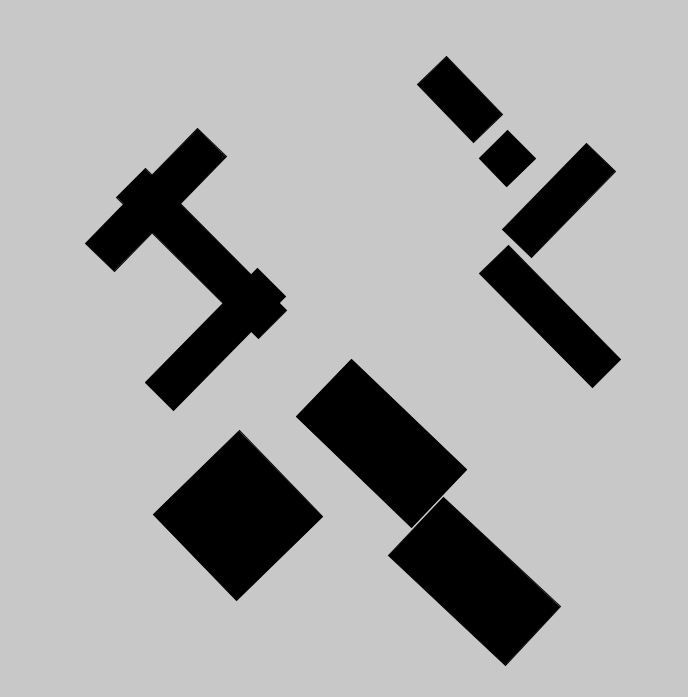### sketch_2023_01_09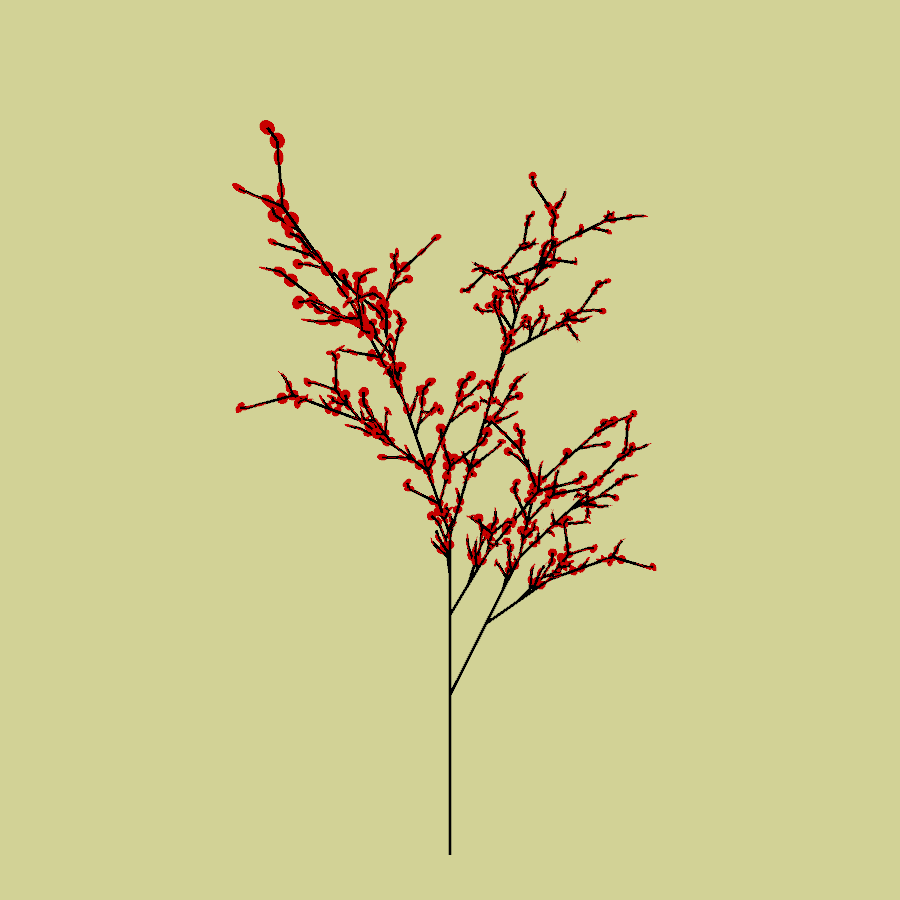### sketch_2023_01_10### sketch_2023_01_08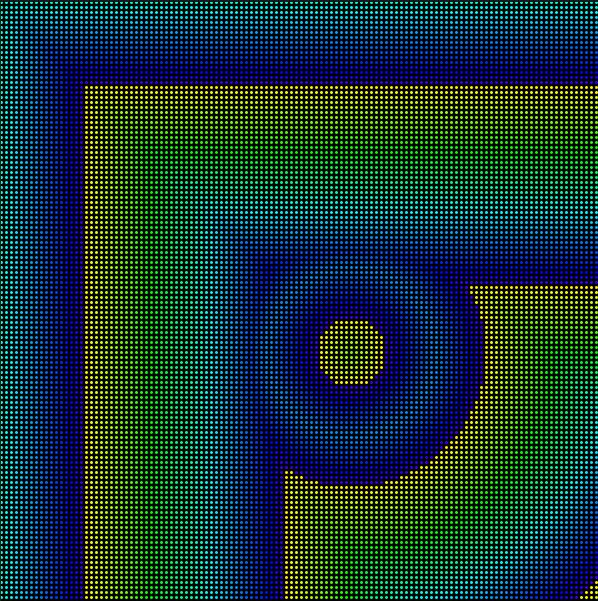### sketch_2023_01_07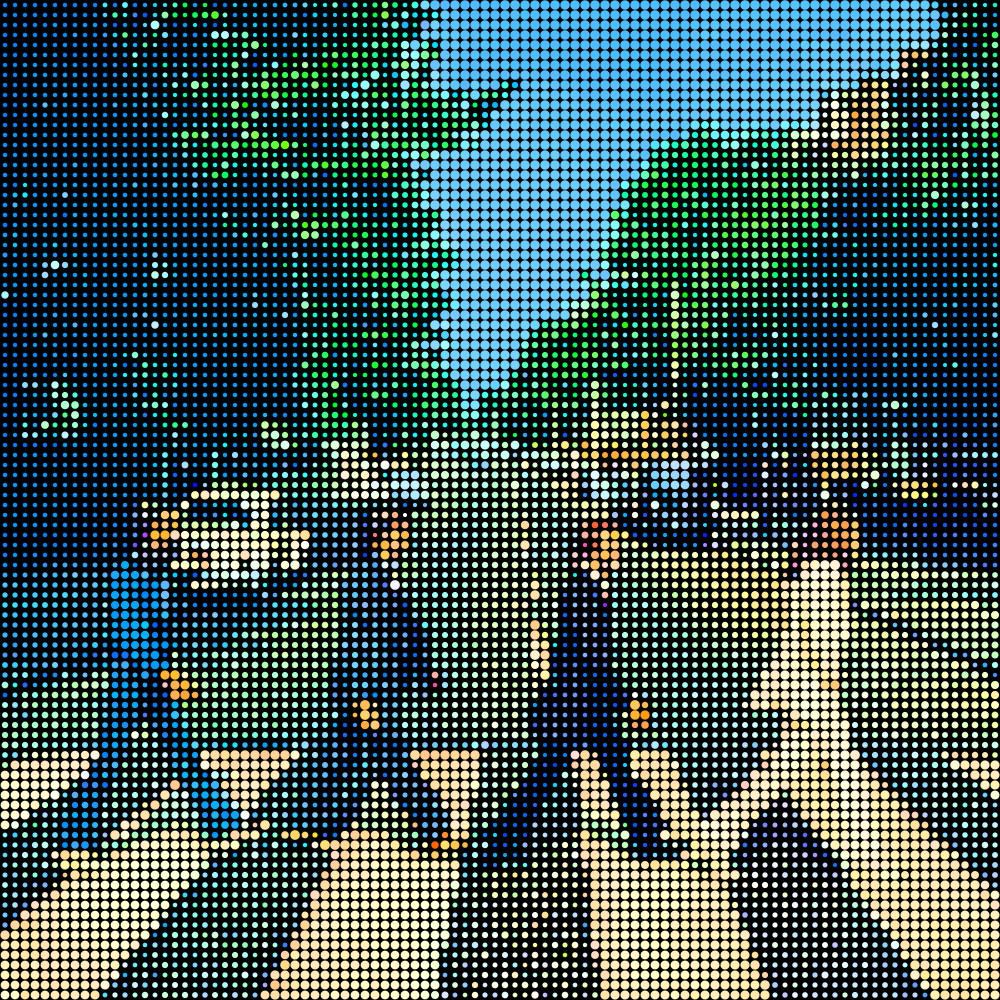``````"""
A reticule of colored circles forms The Beatles's Abbey Road album cover
"""
import py5

def setup():
py5.size(1000, 1000)
py5.color_mode(py5.HSB)
py5.no_stroke()
py5.background(0)
s = 10
w = py5.width // s
for i in range(w):
for j in range(w):
x, y = s // 2 + i * s, s // 2 + j * s
px = img.get(x, y)
h = py5.hue(px)
sat = py5.saturation(px)
b = 0.36 + py5.brightness(px) / 400
py5.fill(h, sat * 2, 255)
py5.circle(x, y, s * b)
py5.save('out.png')

py5.run_sketch()
``````

### sketch_2023_01_06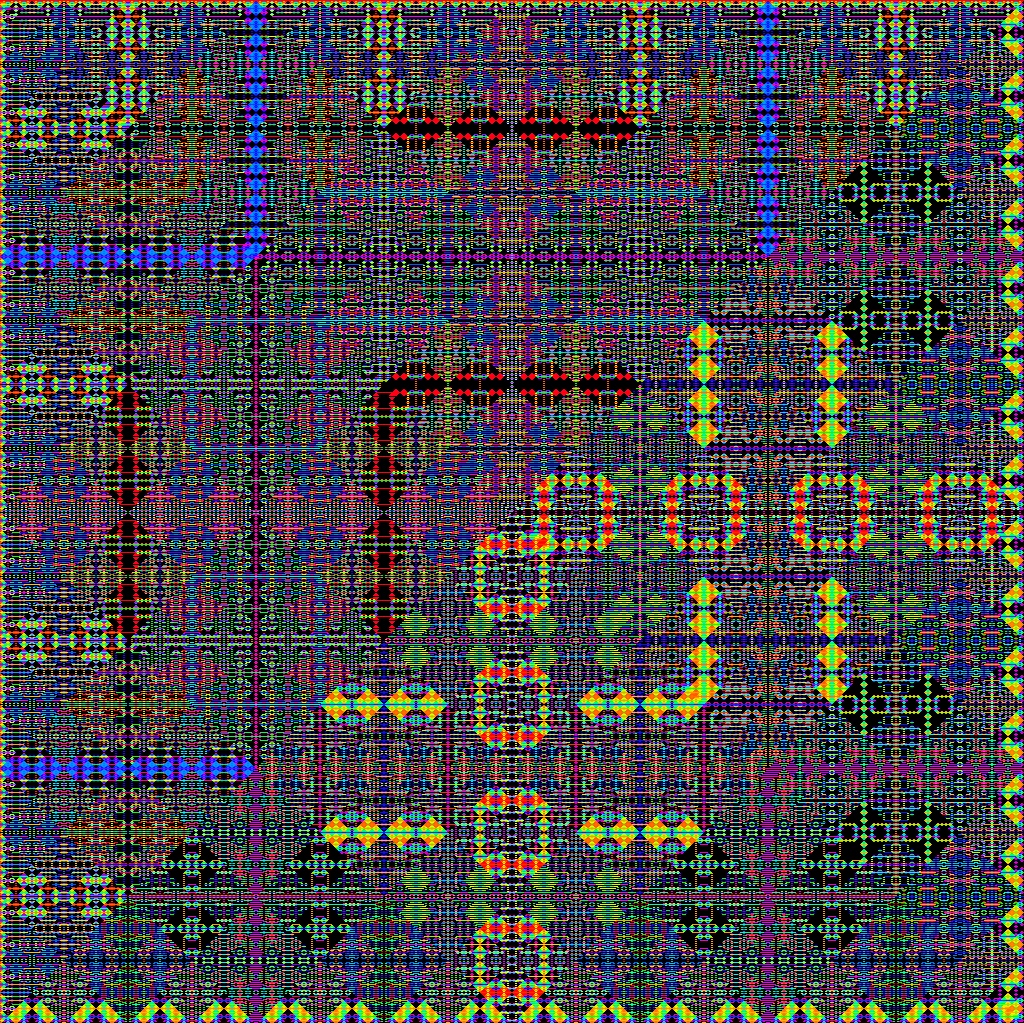### sketch_2023_01_05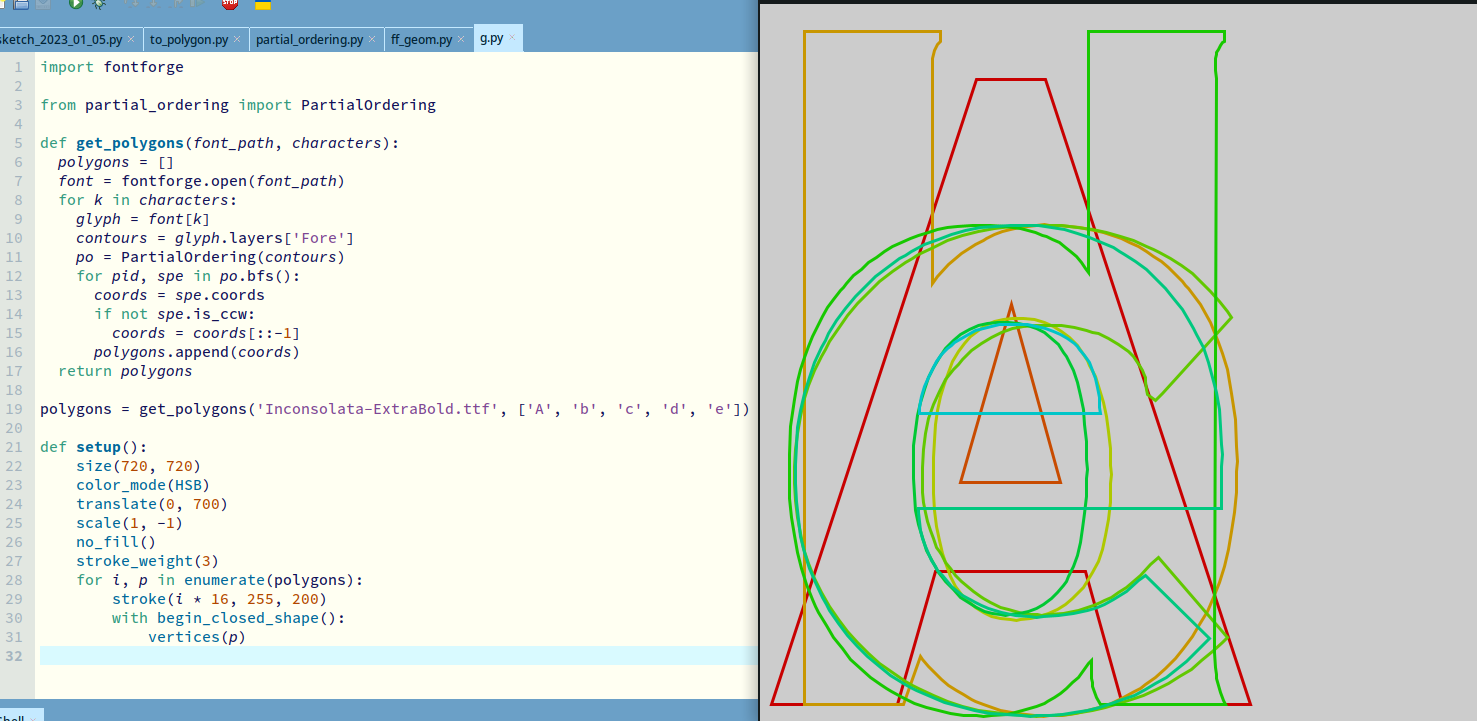### sketch_2023_01_04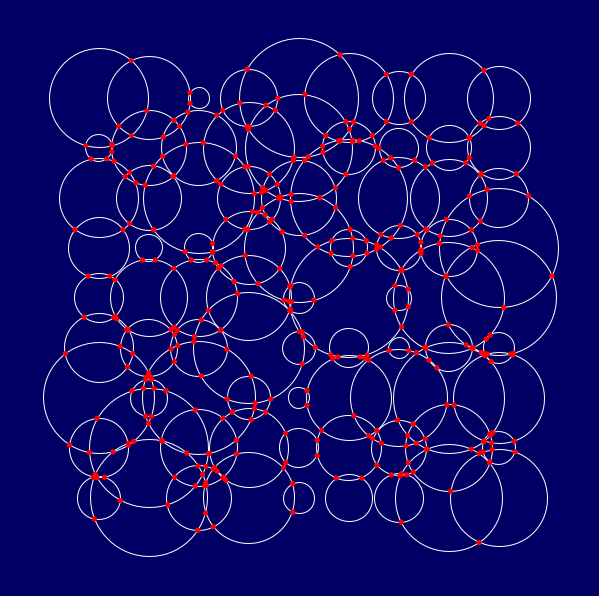``````from itertools import product

circles = []
intersections = []

def setup():
size(600, 600)
for x, y in product(range(100, 550, 50), repeat=2):
r = random(10, 60)
circles.append((x, y, r))
for xa, ya, ra in circles:
for xb, yb, rb in circles[1:]:
if (xa, ya) != (xb, yb):
pts = calc_cci(xa, ya, ra, xb, yb, rb)
intersections.extend(pts)
background(0, 0, 100)
stroke(255)
no_fill()
for x, y, r in circles:
circle(x, y, r * 2)
no_stroke()
fill(255, 0, 0)
for x, y in intersections:
circle(x, y, 5)

def calc_cci(x0, y0, r0, x1 ,y1, r1):
d = dist(x0, y0, x1, y1)
a = (r0 ** 2-r1 ** 2+d ** 2) / (2 * d)
hsqd = r0 ** 2 - a ** 2
if hsqd == 0:
return [(x0 + a * (x1 - x0) / d, y0 + a * (y1 - y0) / d)]
elif hsqd > 0:
h = sqrt(hsqd)
x2 = x0 + a * (x1 - x0) / d
y2 = y0 + a * (y1 - y0) / d
xa, ya = x2 + h * (y1 - y0) / d, y2 - h * (x1 - x0) / d
xb, yb = x2 - h * (y1 - y0) / d, y2 + h * (x1 - x0) / d
return[(xa, ya), (xb, yb)]
else:
return []
``````

### sketch_2023_01_03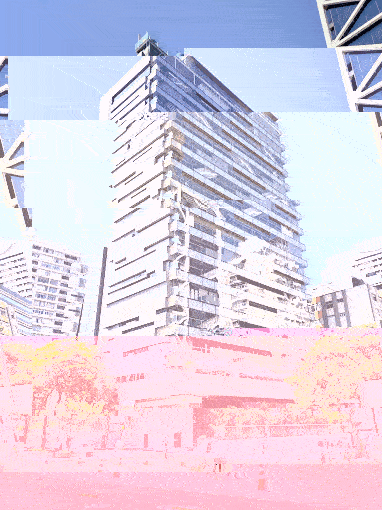Java version:

``````// Para criar imagem com glitch a partir de
// arquivo JPG na sub-pasta /data

PImage img;
int n = 10;  // número de passos estragando bytes!
String nomeArquivo = "sesc.jpg";

void setup() {
size(382, 510);
noLoop();
}

void draw() {
byte[] data=loadBytes(nomeArquivo);    // carrega a imagem original
for (int i = 0; i < n; i++) {
int loc=(int)random(1, data.length);  // sorteia uma posição no array
data[loc]=(byte)random(255);          // sorteia um valor de byte e substitui
}
saveBytes("gliched_" + nomeArquivo, data);         // salva um novo arquivo modificado
image (img, 0, 0);
}

void keyPressed() {
saveFrame("###.png");
redraw();
}
``````

Python version:

``````# using py5 (py5coding.org) imported mode

from PIL import Image
import io

def setup():
size(517, 707)
no_loop()

def draw():
with open('data/a.jpg', 'rb') as f:

for _ in range(10):
loc = random_int(len(list_jpg_bytes) - 1)
list_jpg_bytes[loc] = random_int(1, 255)

stream = io.BytesIO(bytes(list_jpg_bytes))
new_img = Image.open(stream)
try:
image(convert_image(new_img), 0, 0) # PIL.Image to py5Image
except: # OSError / UnidentifiedImageError:
pass

def key_pressed():
save_frame("###.png");
redraw()
``````

### sketch_2023_01_02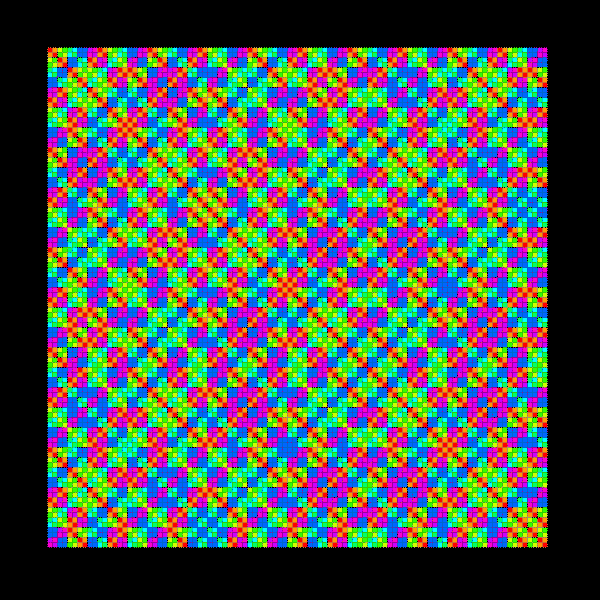Genuary 2 - 10 minutes

``````def setup():
size(600, 600)
color_mode(HSB)
no_stroke()
rect_mode(CENTER)

def draw():
background(0)
m = frame_count #1 + mouse_x // 10
for x in range(100):
cx = 50 + x * 5
for y in range(100):
cy = 50 + y * 5
c = (x ^ y) ** 13 % m
fill(c * (255 / m), 255, 255)
#             print(c, cx, cy)
square(cx, cy, 5)

if frame_count % 10 == 0 and frame_count <= 100:
save_frame('###.png')
``````

### sketch_2023_01_01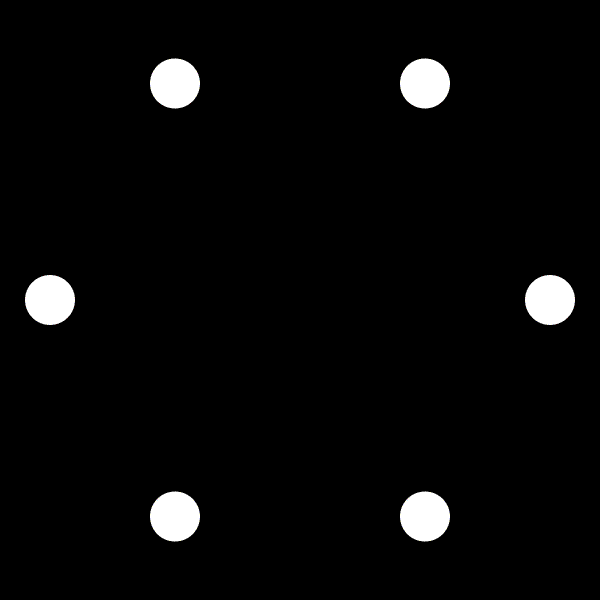Genuary 1 - loop

``````def setup():
size(600, 600)
no_stroke()

def draw():
background(0)
xc = yc = 300
r = 250
for i in range(6):
m = cos(radians(frame_count / 2)) ** 2
a = radians(frame_count / 2 + 60 * i)
x = xc + r * cos(a)
y = yc + r * sin(a)
fill(0, 0, 255)
circle(x, y, 50)
x = xc + r * cos(a * m)
y = yc + r * sin(a * m)
fill(0, 255, 0)
circle(x, y, 50)
x = xc + r * cos(a * -m)
y = yc + r * sin(a * -m)
fill(255, 0, 0)
circle(x, y, 50)
``````

## 2023 | 2022 | 2021 | 2020 | 2019 | 2018

The sketch-a-day project, images and code repository, by Alexandre B A VIllares are licensed under Attribution-NonCommercial-NoDerivatives 4.0 International, except if marked/attributed otherwise in a file or code section. Please contact for licensing questions.